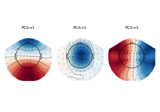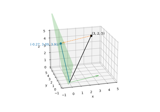# mne.Projection¶

class mne.Projection[source]

Projection vector.

A basic class to proj a meaningful print for projection vectors.

Methods

 __contains__(key, /) True if the dictionary has the specified key, else False. __getitem__ x.__getitem__(y) <==> x[y] Implement iter(self). Return len(self). fromkeys(iterable[, value]) Create a new dictionary with keys from iterable and values set to value. get(key[, default]) Return the value for key if key is in the dictionary, else default. plot_topomap(info[, cmap, sensors, …]) Plot topographic maps of SSP projections. pop(k[,d]) If key is not found, d is returned if given, otherwise KeyError is raised Remove and return a (key, value) pair as a 2-tuple. setdefault(key[, default]) Insert key with a value of default if key is not in the dictionary. update([E, ]**F) If E is present and has a .keys() method, then does: for k in E: D[k] = E[k] If E is present and lacks a .keys() method, then does: for k, v in E: D[k] = v In either case, this is followed by: for k in F: D[k] = F[k]
__contains__(key, /)

True if the dictionary has the specified key, else False.

__getitem__()

x.__getitem__(y) <==> x[y]

__iter__(/)

Implement iter(self).

__len__(/)

Return len(self).

clear() → None. Remove all items from D.
copy() → a shallow copy of D
fromkeys(iterable, value=None, /)

Create a new dictionary with keys from iterable and values set to value.

get(key, default=None, /)

Return the value for key if key is in the dictionary, else default.

items() → a set-like object providing a view on D’s items
keys() → a set-like object providing a view on D’s keys
plot_topomap(info, cmap=None, sensors=True, colorbar=False, res=64, size=1, show=True, outlines='head', contours=6, image_interp='bilinear', axes=None, vlim=(None, None), sphere=None, border='mean')[source]

Plot topographic maps of SSP projections.

Parameters
infoinstance of Info | None

The measurement information to use to determine the layout.

cmapmatplotlib colormap | (colormap, bool) | ‘interactive’ | None

Colormap to use. If tuple, the first value indicates the colormap to use and the second value is a boolean defining interactivity. In interactive mode (only works if colorbar=True) the colors are adjustable by clicking and dragging the colorbar with left and right mouse button. Left mouse button moves the scale up and down and right mouse button adjusts the range. Hitting space bar resets the range. Up and down arrows can be used to change the colormap. If None (default), ‘Reds’ is used for all positive data, otherwise defaults to ‘RdBu_r’. If ‘interactive’, translates to (None, True).

sensors

Add markers for sensor locations to the plot. Accepts matplotlib plot format string (e.g., ‘r+’ for red plusses). If True, a circle will be used (via .add_artist). Defaults to True.

colorbarbool

Plot a colorbar.

resint

The resolution of the topomap image (n pixels along each side).

sizescalar

Side length of the topomaps in inches (only applies when plotting multiple topomaps at a time).

showbool

Show figure if True.

outlines‘head’ | ‘skirt’ | dict | None

The outlines to be drawn. If ‘head’, the default head scheme will be drawn. If ‘skirt’ the head scheme will be drawn, but sensors are allowed to be plotted outside of the head circle. If dict, each key refers to a tuple of x and y positions, the values in ‘mask_pos’ will serve as image mask. Alternatively, a matplotlib patch object can be passed for advanced masking options, either directly or as a function that returns patches (required for multi-axis plots). If None, nothing will be drawn. Defaults to ‘head’.

contours

The number of contour lines to draw. If 0, no contours will be drawn. When an integer, matplotlib ticker locator is used to find suitable values for the contour thresholds (may sometimes be inaccurate, use array for accuracy). If an array, the values represent the levels for the contours. Defaults to 6.

image_interpstr

The image interpolation to be used. All matplotlib options are accepted.

axesinstance of Axes | list | None

The axes to plot to. If list, the list must be a list of Axes of the same length as the number of projectors. If instance of Axes, there must be only one projector. Defaults to None.

vlimtuple of length 2 | ‘joint’

Colormap limits to use. If tuple, specifies the lower and upper bounds of the colormap (in that order); providing None for either of these will set the corresponding boundary at the min/max of the data (separately for each projector). The keyword value 'joint' will compute the colormap limits jointly across all provided projectors of the same channel type, using the min/max of the projector data. If vlim is 'joint', info must not be None. Defaults to (None, None).

sphere

The sphere parameters to use for the cartoon head. Can be array-like of shape (4,) to give the X/Y/Z origin and radius in meters, or a single float to give the radius (origin assumed 0, 0, 0). Can also be a spherical ConductorModel, which will use the origin and radius. Can be “auto” to use a digitization-based fit. Can also be None (default) to use ‘auto’ when enough extra digitization points are available, and 0.095 otherwise. Currently the head radius does not affect plotting.

New in version 0.20.

borderfloat | ‘mean’

Value to extrapolate to on the topomap borders. If 'mean' (default), then each extrapolated point has the average value of its neighbours.

New in version 0.20.

Returns
figinstance of Figure

Figure distributing one image per channel across sensor topography.

Notes

New in version 0.15.0.

Examples using plot_topomap:pop(k[, d]) → v, remove specified key and return the corresponding value.

If key is not found, d is returned if given, otherwise KeyError is raised

popitem(/)

Remove and return a (key, value) pair as a 2-tuple.

Pairs are returned in LIFO (last-in, first-out) order. Raises KeyError if the dict is empty.

setdefault(key, default=None, /)

Insert key with a value of default if key is not in the dictionary.

Return the value for key if key is in the dictionary, else default.

update([E, ]**F) → None. Update D from dict/iterable E and F.

If E is present and has a .keys() method, then does: for k in E: D[k] = E[k] If E is present and lacks a .keys() method, then does: for k, v in E: D[k] = v In either case, this is followed by: for k in F: D[k] = F[k]

values() → an object providing a view on D’s values

## Examples using mne.Projection¶